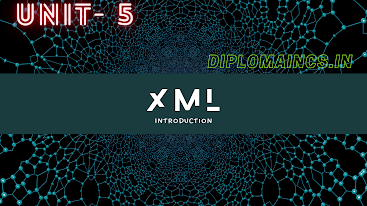## 7/15/20

### Computer programming through C 2018 question

Sub Code: - 1600302

2018(Odd)

Time : 3Hrs

Sem. III(New)

CPTC(CSE)

Full Marks : 70

Pass marks : 28

Group A

Choose the most suitable answer from the following options: -   (1*20=20)

(i) "C" language has been developed by:-
(a) James Gosling
(b) Bjarne Stroustrup
(c) Dennis Ritchie
(d) Charles Babage

(ii) The "#" of the complier directive must appear at the______ of a line.

(a) Beginning
(b) Middle
(c) End
(d) None of the above

(iii) Which function is used to release the memory allotted in dynamic memory allocation?

(a) Malloc()
(b) Free()
(c) Calloc()
(d) Delete

(iv) The ______ statement cause the loop to be terminated.

(a) Exit
(b) Break
(c) Default
(d) Continue

(v) Which of the following is logical operator?

(a) &&
(b) !=
(c) |
(d) All of the above

(vi) If 'a' is an integer variable, then a=5/2: will return a value

(a) 2.5
(b) 3
(c) 2
(d) 0

(vii) Which of the following is false in 'C'

(a) Capital letters can be used in variable names
(b) Variable names can contain a digit
(c) Variable names do not contain a blank space
(d) keywords can be used as a variable name

(viii) Which of the following is a correct way of declaring pointer:

(a) float ptr;
(b) float *ptr;
(c) *float ptrl;
(d) None of the above;

(ix) Which committee standardize "C" programming language?

(a) ANSI
(b) W3C
(c) TRAI
(d) ISO

(x) Which of the following can not be checked in a switch case statement?

(a) Character
(b) Integer
(c) Float
(d) None of the above

(xi) Which of the statement we need to write, in order to check any condition?

(a) If
(b) Break
(c) Continue
(d) Exit

(xii) Which of the following is not an example of looping statements ?

(a) For
(b) Do-While
(c) While
(d) Switch

(xiii) Which of the following is a bit-wise operator?

(a) <
(b) <<
(c) =
(d) ==

(xiv) Find out the output for the following program : -

main()

{

do

printf("I am in loop");

while(4<3);

}

(a) Error
(b) I am in loop
(c) Compilation error
(d) None of the above

(xv) Find out the output of the following program

main()

{

int x=2,y=3;

printf("%d%d");

}

(a) 2 3
(b) 3 2
(c) compilation error
(d) none of these

(xvi) An 'int' data types requires:-

(a) 2 bytes
(b) 3 bytes
(c) 1 bytes
(d) 8 bytes

(xvii) Find out the output for the following program

main()

{

int x=4;

if(x==2)

printf("you are right");

else

printf("you are wrong")

}

(a) you are right
(b) you are wrong
(c) compilation error
(d) syntax error

(xviii) "!=" represents

(a) Greater
(b) Equal to
(c) Not equal to
(d) Syntax error

(xix) Member of union use:

(a) Different storage location
(b) Same storage locations
(c) No storage locations
(d) None of these

(xx) ASCII value of "A" is:

(a) 65
(b) 32
(c) 97
(d) 38

Group:-"B"

Answer all Five Questions: -                         (5*4=20)

2.  What is library function? ? Mention any two type of library function in C

OR

Write a C program to print "Hello" message using while loop.

3. Explain different data types in "C"

OR

What is the general form of "do-while" statement? Explain with examples.

4. Write a C program to find the sum and average of given three numbers from the user.

OR

Convert the following mathematical expressions into C expression

5. How does "X++" different from "++X"? Explain with suitable example

OR

Explain the syntax of scanf() and printf() functions with suitable example.

6. Write the algorithm and draw the flowchart to ind the sum and product of given two numbers

OR

What is pointer? Give examples

Group:- "C"

Answer all Five Questions: -                         (6*5=30)

7. Write a C program to find the biggest of given two numbers.

OR

Explain the various if structure in C language.

8. Write down the general syntax of switch statement and explain with the help of the examples.

OR

Write a program to find a square root of quadratic equation.

9. Write a C program to print natural number from 1 to n.

OR

What do you mean by an algorithm?  Write an algorithm of finding area of triangle.

10. Explain different types of loop control structure used in C language.

OR

Write a C program for addition of two matrix.

11. Explain the various types of function supported by C. give example for each of the C functions

OR

What is a structure? How it is different from an array? How are they defined and initialized? Explain with example.

(Note:- Update available soon, comment for any type of help

NOTES of ISP (unit-4): -

}

#### 1 comment:

1.